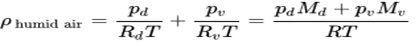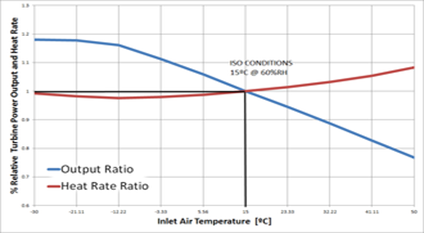• info@aquachill.co.in
• +91 9922503151Turbine inlet air cooling is a group of technologies and techniques consisting of cooling down the intake air of the gas turbine. The direct consequence of cooling the turbine inlet air is power output augmentation. It may also improve the energy efficiency of the system. This technology is widely used in hot climates with high ambient temperatures that usually coincides with on-peak demand period.

## Principles

Gas turbines take in filtered, fresh ambient air and compress it in the compressor stage. The compressed air is mixed with fuel in the combustion chamber and ignited. This produces a high-temperature and high-pressure flow of exhaust gases that enter in a turbine and produce the shaft work output that is generally used to turn an electric generator as well as powering the compressor stage.

As the gas turbine is a constant volume machine, the air volume introduced in the combustion chamber after the compression stage is fixed for a given shaft speed (rpm). Thus, the air mass flow in is directly related to the density of air, and the introduced volume.

Mathematical presentation/formulation:

The air mass flow in is directly related to the density of air, and the introduced volume.

m=ρV

whereis the mass,is the density andis the volume of the gas. As the volumeis fixed, only densityof the air can be modified to vary air mass. The density of the air depends of the relative humidity, altitude, pressure drop and temperature.Where,

ρ humid air = Density of the humid air (kg/m³)

ρd = Partial pressure of dry air (Pa)

Specific gas constant for dry air, 287.058 J/ (kg· K)

T   = Temperature (K)

ρv = Pressure of water vapor (Pa)

Rv = Specific gas constant for water vapor, 461.495 J/ (kg· K)

Md = Molar mass of dry air, 0.028964 (kg/mol)

Mv = Molar mass of water vapor, 0.018016 (kg/mol)

R = , 8.314 J/ (K· mol)

The performance of a gas turbine, its efficiency (heat rate) and the generated power output strongly depend on the climate conditions, which may decrease the output power ratings by up to 40%. To operate the turbine at ISO conditions and recover performance, several inlet air cooling systems have been promoted.## Recent Post

•#### Effective ways of Duct Design (New)

8 months ago
•#### Energy Efficiency in Primary Variable System

8 months ago
•#### Inlet Air – Cooling System of a Gas Turbine

8 months ago
•